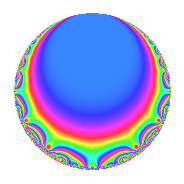# Properties

 Label 8001.2.a.iLevel 8001 Weight 2 Character orbit 8001.a Self dual Yes Analytic conductor 63.888 Analytic rank 1 Dimension 2 CM No Inner twists 1

# Related objects

## Newspace parameters

 Level: $$N$$ = $$8001 = 3^{2} \cdot 7 \cdot 127$$ Weight: $$k$$ = $$2$$ Character orbit: $$[\chi]$$ = 8001.a (trivial)

## Newform invariants

 Self dual: Yes Analytic conductor: $$63.8883066572$$ Analytic rank: $$1$$ Dimension: $$2$$ Coefficient field: $$\Q(\sqrt{17})$$ Coefficient ring: $$\Z[a_1, \ldots, a_{5}]$$ Coefficient ring index: $$1$$ Fricke sign: $$1$$ Sato-Tate group: $\mathrm{SU}(2)$

## $q$-expansion

Coefficients of the $$q$$-expansion are expressed in terms of $$\beta = \frac{1}{2}(1 + \sqrt{17})$$. We also show the integral $$q$$-expansion of the trace form.

 $$f(q)$$ $$=$$ $$q$$ $$-2 q^{4}$$ $$+ ( 1 + \beta ) q^{5}$$ $$- q^{7}$$ $$+O(q^{10})$$ $$q$$ $$-2 q^{4}$$ $$+ ( 1 + \beta ) q^{5}$$ $$- q^{7}$$ $$+ ( 2 - 2 \beta ) q^{11}$$ $$+ ( -1 - \beta ) q^{13}$$ $$+ 4 q^{16}$$ $$+ ( -4 - \beta ) q^{17}$$ $$+ 4 q^{19}$$ $$+ ( -2 - 2 \beta ) q^{20}$$ $$+ ( -3 + 3 \beta ) q^{23}$$ $$+ 3 \beta q^{25}$$ $$+ 2 q^{28}$$ $$+ ( 1 - 2 \beta ) q^{29}$$ $$+ ( -3 + 3 \beta ) q^{31}$$ $$+ ( -1 - \beta ) q^{35}$$ $$+ ( -7 + 2 \beta ) q^{37}$$ $$+ ( -2 + 5 \beta ) q^{41}$$ $$+ ( 6 - 4 \beta ) q^{43}$$ $$+ ( -4 + 4 \beta ) q^{44}$$ $$+ ( 10 - 2 \beta ) q^{47}$$ $$+ q^{49}$$ $$+ ( 2 + 2 \beta ) q^{52}$$ $$+ 11 q^{53}$$ $$+ ( -6 - 2 \beta ) q^{55}$$ $$+ ( 7 - \beta ) q^{59}$$ $$+ ( -7 - \beta ) q^{61}$$ $$-8 q^{64}$$ $$+ ( -5 - 3 \beta ) q^{65}$$ $$-2 q^{67}$$ $$+ ( 8 + 2 \beta ) q^{68}$$ $$+ ( 8 + 2 \beta ) q^{71}$$ $$+ ( -7 + \beta ) q^{73}$$ $$-8 q^{76}$$ $$+ ( -2 + 2 \beta ) q^{77}$$ $$+ ( -8 + 3 \beta ) q^{79}$$ $$+ ( 4 + 4 \beta ) q^{80}$$ $$+ ( -5 - 5 \beta ) q^{83}$$ $$+ ( -8 - 6 \beta ) q^{85}$$ $$+ ( 5 - 5 \beta ) q^{89}$$ $$+ ( 1 + \beta ) q^{91}$$ $$+ ( 6 - 6 \beta ) q^{92}$$ $$+ ( 4 + 4 \beta ) q^{95}$$ $$+ ( 4 - 5 \beta ) q^{97}$$ $$+O(q^{100})$$ $$\operatorname{Tr}(f)(q)$$ $$=$$ $$2q$$ $$\mathstrut -\mathstrut 4q^{4}$$ $$\mathstrut +\mathstrut 3q^{5}$$ $$\mathstrut -\mathstrut 2q^{7}$$ $$\mathstrut +\mathstrut O(q^{10})$$ $$2q$$ $$\mathstrut -\mathstrut 4q^{4}$$ $$\mathstrut +\mathstrut 3q^{5}$$ $$\mathstrut -\mathstrut 2q^{7}$$ $$\mathstrut +\mathstrut 2q^{11}$$ $$\mathstrut -\mathstrut 3q^{13}$$ $$\mathstrut +\mathstrut 8q^{16}$$ $$\mathstrut -\mathstrut 9q^{17}$$ $$\mathstrut +\mathstrut 8q^{19}$$ $$\mathstrut -\mathstrut 6q^{20}$$ $$\mathstrut -\mathstrut 3q^{23}$$ $$\mathstrut +\mathstrut 3q^{25}$$ $$\mathstrut +\mathstrut 4q^{28}$$ $$\mathstrut -\mathstrut 3q^{31}$$ $$\mathstrut -\mathstrut 3q^{35}$$ $$\mathstrut -\mathstrut 12q^{37}$$ $$\mathstrut +\mathstrut q^{41}$$ $$\mathstrut +\mathstrut 8q^{43}$$ $$\mathstrut -\mathstrut 4q^{44}$$ $$\mathstrut +\mathstrut 18q^{47}$$ $$\mathstrut +\mathstrut 2q^{49}$$ $$\mathstrut +\mathstrut 6q^{52}$$ $$\mathstrut +\mathstrut 22q^{53}$$ $$\mathstrut -\mathstrut 14q^{55}$$ $$\mathstrut +\mathstrut 13q^{59}$$ $$\mathstrut -\mathstrut 15q^{61}$$ $$\mathstrut -\mathstrut 16q^{64}$$ $$\mathstrut -\mathstrut 13q^{65}$$ $$\mathstrut -\mathstrut 4q^{67}$$ $$\mathstrut +\mathstrut 18q^{68}$$ $$\mathstrut +\mathstrut 18q^{71}$$ $$\mathstrut -\mathstrut 13q^{73}$$ $$\mathstrut -\mathstrut 16q^{76}$$ $$\mathstrut -\mathstrut 2q^{77}$$ $$\mathstrut -\mathstrut 13q^{79}$$ $$\mathstrut +\mathstrut 12q^{80}$$ $$\mathstrut -\mathstrut 15q^{83}$$ $$\mathstrut -\mathstrut 22q^{85}$$ $$\mathstrut +\mathstrut 5q^{89}$$ $$\mathstrut +\mathstrut 3q^{91}$$ $$\mathstrut +\mathstrut 6q^{92}$$ $$\mathstrut +\mathstrut 12q^{95}$$ $$\mathstrut +\mathstrut 3q^{97}$$ $$\mathstrut +\mathstrut O(q^{100})$$

## Embeddings

For each embedding $$\iota_m$$ of the coefficient field, the values $$\iota_m(a_n)$$ are shown below.

For more information on an embedded modular form you can click on its label.

Label $$\iota_m(\nu)$$ $$a_{2}$$ $$a_{3}$$ $$a_{4}$$ $$a_{5}$$ $$a_{6}$$ $$a_{7}$$ $$a_{8}$$ $$a_{9}$$ $$a_{10}$$
1.1
 −1.56155 2.56155
0 0 −2.00000 −0.561553 0 −1.00000 0 0 0
1.2 0 0 −2.00000 3.56155 0 −1.00000 0 0 0
 $$n$$: e.g. 2-40 or 990-1000 Significant digits: Format: Complex embeddings Normalized embeddings Satake parameters Satake angles

## Inner twists

This newform does not admit any (nontrivial) inner twists.

## Atkin-Lehner signs

$$p$$ Sign
$$3$$ $$-1$$
$$7$$ $$1$$
$$127$$ $$-1$$

## Hecke kernels

This newform can be constructed as the intersection of the kernels of the following linear operators acting on $$S_{2}^{\mathrm{new}}(\Gamma_0(8001))$$:

 $$T_{2}$$ $$T_{5}^{2}$$ $$\mathstrut -\mathstrut 3 T_{5}$$ $$\mathstrut -\mathstrut 2$$